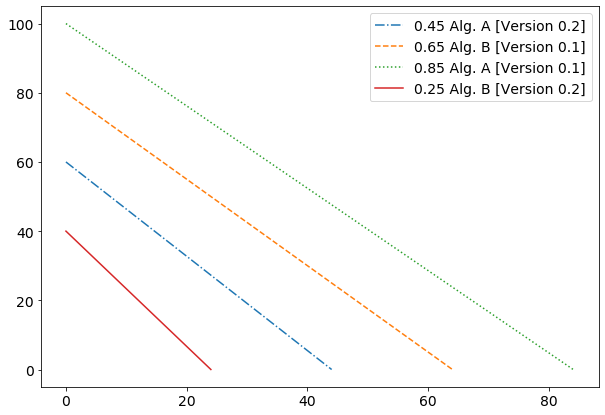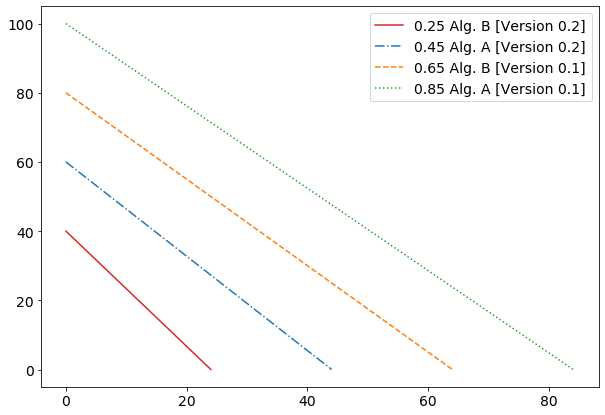# Decorate-Sort-Undecorate: Advanced list sorting in Python

Tags:

Categories:

Updated:

## Motivation and use case

The other day, I found myself generating a complex performance graph using matplotlib. I wanted to rearrange the order of the item in the legend. There are multiple ways we could tackle this problem: We could, for example, manipulate the order in which the items are plotted since this effects the order in which the legend data is generated. The, in my opinion, much more elegant way is to directly manipulate the order of the items in matplotlib’s legend. Since the legend consists of two lists (a list of handles and a list of labels), I needed a solution for sorting both lists.

## Simple example: Sorting two lists in the same manner

We will start simple and, for now, only consider sorting two lists (that indirectly reference each other) in the same order. The more complex example, where we sort two lists by the order of a third list, will be introduced in the following section.

Consider the following example code:

import numpy as np
import matplotlib.pyplot as plt
import matplotlib

matplotlib.rcParams.update({'font.size': 14})

# init figurebare
fig, ax = plt.subplots(1,1, figsize=(10,7))

# generate artificial data
line_a = np.linspace(100, 0, 85)
line_b = np.linspace(80, 0, 65)
line_c = np.linspace(60, 0, 45)
line_d = np.linspace(40, 0, 25)

# plot artificial data
plt.plot(line_d, label="0.25 Alg. B [Version 0.2]")
plt.plot(line_c, ls="-.", label="0.45 Alg. A [Version 0.2]")
plt.plot(line_b, ls="--", label="0.65 Alg. B [Version 0.1]")
plt.plot(line_a, ls=":", label="0.85 Alg. A [Version 0.1]")
plt.legend()
plt.show()


This code produces the following plot:Pay close attention to the legend! There appears to be no ordering of the legend entries. We can observe that each item in the plot (the four lines) is identified by it’s linestyle and has a describing text, indicating a performance measure. These are the two lists that I mentioned earlier: The legend handles are the linestyle elements, and the labels are the pieces of text describing each item in the plot.

We can get these two lists using the following command :

# handles and labels are of type list
handles, labels = ax.get_legend_handles_labels()


We don’t have to worry too much about how the handles list looks like since we don’t want to sort by the linestyle. Instead, we want to sort the legend entries according to the text, describing each item in the plot. Thus, let’s inspect the labels list:

labels
['0.45 Alg. A [Version 0.2]',
'0.65 Alg. B [Version 0.1]',
'0.85 Alg. A [Version 0.1]',
'0.25 Alg. B [Version 0.2]',]


Sticking to the simple case, let’s fix the weird-looking ordering of the items in the legend. Luckily, Python makes it easy to sort two lists in the same way! It should be clear by now why we need to sort both lists: If we only sort the list of the labels, they will no longer match their handle! This means, that the text in the legend would no longer match its preceding linestyle! This is very dangerous and should never be done, since it manipulates the entire plot! Thus, the following code sorts both lists according to the items in the first list:

ordered_labels, ordered_handles = zip(*sorted(zip(labels, handles)))
ordered_labels
('0.25 Alg. B [Version 0.2]',
'0.45 Alg. A [Version 0.2]',
'0.65 Alg. B [Version 0.1]',
'0.85 Alg. A [Version 0.1]')


Here, we already (implicitly) made use of the ‘Decorate-Sort-Undecorate’ idiom, but more on that later. Let’s break the ordered_labels, ordered_handles = zip(*sorted(zip(labels, handles))) line down into smaller pieces, to really understand what’s happening. The most inner zip(labels, handles) shouldn’t be too mysterious. The zip() function simply does what it always does: Creating an iterator of n-tuples (meaning there are n elements in the tuple), depending on how many iterable we pass into zip() function. To give an example:

for tuple in zip(["fgh", "asd"], ["456", "123"]):
print(tuple)
('fgh', '456')
('asd', '123')


In our case, this generates an iterator where each tuple contains one element of both lists, meaning the i-th tuple contains the i-th legend handle and i-th legend label. So far, so good, but what does the *sorted(...) do? Well, sorted simply sorts the items of a given iterable (the iterable we get from the most inner zip().

sorted(zip(["fgh", "asd"], ["456", "123"]))
[('fgh', '456'), ('asd', '123')]


Now, we get a list of sorted tuples. But we want two sorted lists! Here, the asterisk * comes into play. The asterisk, in this case, simply unpacks the list it receives as input into its positional arguments.

print(*sorted(zip(["fgh", "asd"], ["456", "123"])))
('asd', '123') ('fgh', '456')


So now we no longer have a list of tuples, but rather an iterable of tuples. Remember what we could do with an iterable? Throw the iterable at zip() and get an iterable of n-tuples back! See where this is going? Look at the following snippet:

for tuple in zip(*sorted(zip(["fgh", "asd"], ["456", "123"]))):
print(tuple)
('asd', 'fgh')
('123', '456')


This is exactly what we wanted! Both lists have been sorted according to the items in the first list (actually, these are now tuples and not lists, but you can cast them into a list if it matters in your use case). Applying this to the initial snipped produces the following plot (if you want to take a look the complete, update snipped Decorate-Sort-Undecorate, also known as the here is the Github gist):

Here is the resulting plot, in which the legend entries are sorted by the performance measure value:The legend looks much better! The entries in the legend are sorted in an ascending manner regarding the performance measure. But, what if we aren’t yet happy with the way the entries in the legend are ordered? What, if we don’t want to sort by the performance measure, but instead by the algorithm name or the version of each algorithm? This, we will explore in the next two sections, so hold on to your coffee mugs and bear with me!

## The 'Decorate-Sort-Undecorate' idiom

So what is this mysterious ‘Decorate-Sort-Undecorate’ idiom, which is dominantly mentioned in the title of this post but has only been used implicitly and without great explanation? The Decorate-Sort-Undecorate, also known as the Schwartzian transform is a technique for

...comparison-based sorting when the ordering is actually based on the ordering of a certain property (the key) of the elements... wikipedia

and has been around since 1994. The idiom gets its name from the three main steps:

1. Create a list (decorate an existing list) with specific values, whose purpose is to control the sorting behavior.
2. Sort the decorated list (possibly apply sorting to another list).
3. Remove decorations from the decorated list (can be ignored if dedicated list with decorated values has been created).

Thus, this idiom describes how to sort a list, when we are not happy with the default sorting behavior. In the next and final section of this post, we will see the idiom in action to apply a custom sorting to our matplotlib legend.

## Advanced example: Sorting two lists by the order of a third

Now, towards the more general example. Say we have two lists, like the list of handles and labels in a matplotlib legend, and we want to sort both of those lists according to some custom sorting behavior. Here, we fully embrace the decorate part of the ‘Decorate-Sort-Undecorate’ idiom by creating an additional list, with the sole purpose of generating the sorting behavior for our two actual lists.

For this, we must first generate the decorated list (the original idiom manipulates the actual list, but I feel like doing this externally is much more intuitive). As mentioned above, let’s say we want to sort our legend entries not by the performance measure, but by the name of the algorithms;

decorated = [text[5:] for text in labels]
decorate
['Alg. A [Version 0.2]',
'Alg. B [Version 0.1]',
'Alg. A [Version 0.1]',
'Alg. B [Version 0.2]']


In the next step, we generate an index list, and sort the indices according to the decorated list.

sorted_indices = list(range(len(decorated)))
sorted_indices.sort(key=decorated.__getitem__)
sorted_indices
[2, 0, 1, 3]


The final step is to simply map the sorted indices to the two lists we want to sort and we are done!

sorted_labels = list(map(labels.__getitem__, sorted_indices))
sorted_handels = list(map(handels.__getitem__, sorted_indices))


And that’s it! Here’s our final plot:We have applied the ‘Decorate-Sort-Undecorate’ idiom to sort two lists in the same manner. The final version of the initial snipped, which contains the above steps is available as gist on Github. I hope you learned something from this post, or if not, at least enjoyed reading it as much as I enjoyed writing it.

Cheers,
Finn.

P.S.: This post has been motivated by my answer on stackoverflow regarding the question Is it possible to sort two lists(which reference each other) in the exact same way?